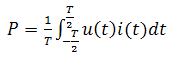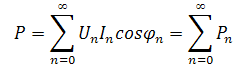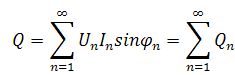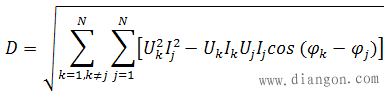# 功率计算公式大全

• A+

## 1、普遍适用的功率计算公式

p(t)=u(t)i(t)   （1）

p(t)=F(t)v(t)  （2）

P=W/T          （3）
W为时间T内做的功。（4）

S=UI

P=UI
P=U2/R
P=I2R

Q=UIsinφ

P=UIcosφ

P2+Q2=S2

Q=0，S=P

## 4、非正弦交流电功率计算公式S2≠P2+Q2

S2=P2+Q2+D2## 6、三相功率计算公式

P=PA+PB+PC

P=PAB+PCB或P=PCA+PBA或P=PBC+PAC

P=√3UIcosφ；U、I为线电压、线电流有效值。

P=3UPIPcosφ；UP、IP为相电压、相电流有效值。

## 7、电机功率计算公式

P=FV   （5）
F：力，单位为N；V：速度，单位为m/s；P：功率，单位为W
T=FR
F=T/R  （6）
T：扭矩，单位为N.m；R：作用半径，单位为m
V=2πRn/60=πR*n/30   （7）
V：线速度，单位为m/s；n：转速，单位为r/min

P=πTn/30

P=πTn/30000=Tn/9549

T=9549P/n

• 营销微信
• 报单询价扫一扫
•• 技术微信
• 技术问题扫一扫
•